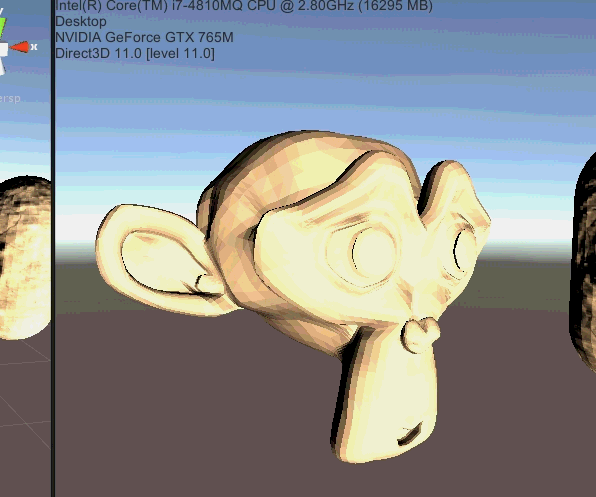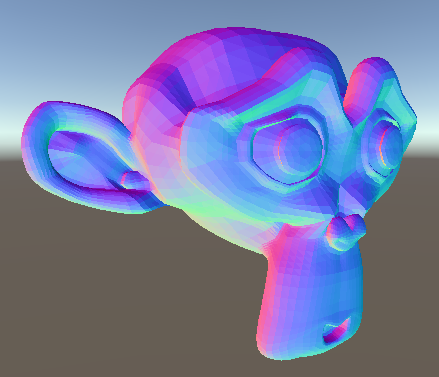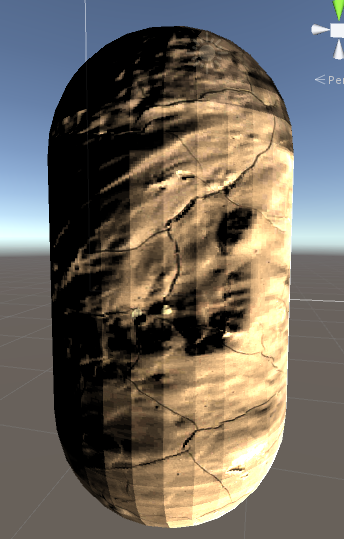# Unity3d ddx ddy 法线x轴 斜率表示前进方向向量， 和 y 轴斜率表示右手方向向量，就可以求 三角面的法线
normalize(ddx) cross normalize(ddy) = normal;
normal = normalize(normal);```			v2f vert (appdata v)
{
v2f o;

float4x4 modelMat = _Object2World;
float4 worldPos = mul(modelMat, v.vertex);

o.worldPos = worldPos;
o.vertex = mul(UNITY_MATRIX_VP, worldPos);
o.uv = v.uv;

return o;
}

fixed4 frag (v2f i) : SV_Target
{
float3 dx = ddx(i.worldPos);
float3 dy = ddy(i.worldPos);
float3 normal = normalize(cross(dx, dy));

normal = -normal * 0.5 + 0.5;
return fixed4(normal,1.0);
}
```

```float3x3 tangleMat = float3x3(normalize(dx), normalize(-dy), normal);
float3 bumpNormal = tex2D(_NormalTex, i.uv);
bumpNormal = bumpNormal * 2 - 1;// range to -1~1 from 0~1
bumpNormal = mul(tangleMat, bumpNormal);
float diff = max(0, dot(i.worldLightDir, bumpNormal));
col *= col * diff;
return fixed4(col,1.0);
```ddx/ddy 还有一个 float2 的参数形式

1. 计算mipmap level 计算当前纹理在屏幕分辨率下的大小是否小于某个mipmap等级的纹理大小，是的话就切换纹理
2. 视差贴图 从视野方向计算像素挤出的位置的uv值
3. and so on…

## 1 评论

1.Li说道：

视差贴图 从视野方向计算像素挤出的位置的uv值

这个能展开讲讲吗

This site uses Akismet to reduce spam. Learn how your comment data is processed.